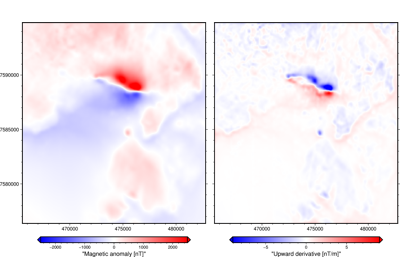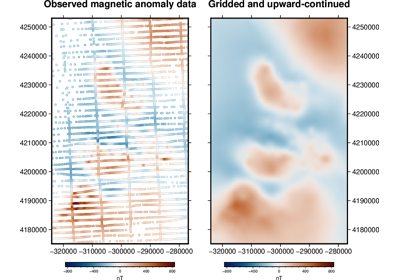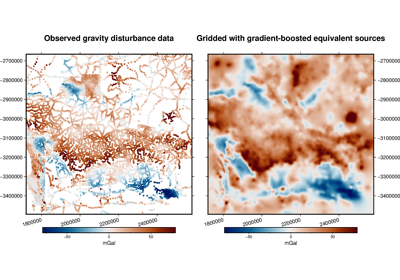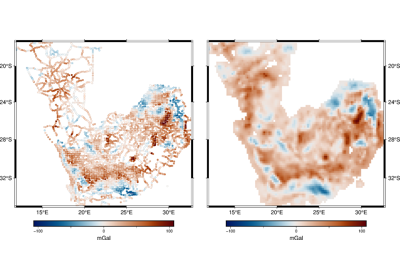# harmonica.EquivalentSources¶

class harmonica.EquivalentSources(damping=None, points=None, depth=500, depth_type='relative', block_size=None, parallel=True, dtype='float64', **kwargs)[source]

Equivalent sources for generic harmonic functions (gravity, magnetics).

These equivalent sources can be used for:

• Cartesian coordinates (geographic coordinates must be project before use)

• Gravity and magnetic data (including derivatives)

• Single data types

• Interpolation

• Upward continuation

• Finite-difference based derivative calculations

They cannot be used for:

• Regional or global data where Earth’s curvature must be taken into account

• Joint inversion of multiple data types (e.g., gravity + gravity gradients)

• Reduction to the pole of magnetic total field anomaly data

• Analytical derivative calculations

By default, the point sources are located beneath the observed potential-field measurement points [Cooper2000] that are passed as arguments to the EquivalentSources.fit method, producing the same number of sources as data points. Alternatively, we can reduce the number of sources by using block-averaged sources [Soler2021]: we divide the data region in blocks of equal size and compute the median location of the observations points that fall under each block. Then, we locate one point source beneath each one of these locations. The size of the blocks, that indirectly controls how many sources will be created, can be specified through the block_size argument. We recommend choosing a block_size no larger than the resolution of the grid where interpolations will be carried out.

The depth of the sources can be controlled by the depth argument. If depth_type is set to "relative", then each source is located beneath each data point or block-averaged location at a depth equal to its elevation minus the value of the depth argument. If depth_type is set to "constant", then every source is located at a constant depth given by the depth argument. In both cases a positive value of depth locates sources _beneath_ the data points or the block-averaged locations, thus a negative depth will put the sources _above_ them.

Custom source locations can be chosen by specifying the points argument, in which case the depth_type, block_size and depth arguments will be ignored.

The corresponding coefficient for each point source is estimated through linear least-squares with damping (Tikhonov 0th order) regularization.

The Green’s function for point mass effects used is the inverse Euclidean distance between the observation points and the point sources:

$\phi(\bar{x}, \bar{x}') = \frac{1}{||\bar{x} - \bar{x}'||}$

where $$\bar{x}$$ and $$\bar{x}'$$ are the coordinate vectors of the observation point and the source, respectively.

Parameters
• damping (None or float) – The positive damping regularization parameter. Controls how much smoothness is imposed on the estimated coefficients. If None, no regularization is used.

• points (None or list of arrays (optional)) – List containing the coordinates of the equivalent point sources. Coordinates are assumed to be in the following order: (easting, northing, upward). If None, will place one point source below each observation point at a fixed relative depth below the observation point [Cooper2000]. Defaults to None.

• depth (float) – Parameter used to control the depth at which the point sources will be located. If depth_type is "constant", each source is located at the same depth specified through the depth argument. If depth_type is "relative", each source is located beneath each data point (or block-averaged location) at a depth equal to its elevation minus the depth value. This parameter is ignored if points is specified. Defaults to 500.

• depth_type (str) – Strategy used for setting the depth of the point sources. The two available strategies are "constant" and "relative". This parameter is ignored if points is specified. Defaults to "relative".

• block_size (float, tuple = (s_north, s_east) or None) – Size of the blocks used on block-averaged equivalent sources. If a single value is passed, the blocks will have a square shape. Alternatively, the dimensions of the blocks in the South-North and West-East directions can be specified by passing a tuple. If None, no block-averaging is applied. This parameter is ignored if points are specified. Default to None.

• parallel (bool) – If True any predictions and Jacobian building is carried out in parallel through Numba’s jit.prange, reducing the computation time. If False, these tasks will be run on a single CPU. Default to True.

• dtype (data-type) – The desired data-type for the predictions and the Jacobian matrix. Default to "float64".

Variables
• points (2d-array) – Coordinates of the equivalent point sources.

• coefs (array) – Estimated coefficients of every point source.

• region (tuple) – The boundaries ([W, E, S, N]) of the data used to fit the interpolator. Used as the default region for the grid method.

References

[Soler2021]

Methods Summary

 EquivalentSources.filter(coordinates, data) Filter the data through the gridder and produce residuals. EquivalentSources.fit(coordinates, data[, ...]) Fit the coefficients of the equivalent sources. Get parameters for this estimator. EquivalentSources.grid(coordinates[, dims, ...]) Interpolate the data onto a regular grid. EquivalentSources.jacobian(coordinates, points) Make the Jacobian matrix for the equivalent sources. EquivalentSources.predict(coordinates) Evaluate the estimated equivalent sources on the given set of points. EquivalentSources.profile(point1, point2, ...) Interpolate data along a profile between two points. EquivalentSources.scatter([region, size, ...]) EquivalentSources.score(coordinates, data[, ...]) Score the gridder predictions against the given data. EquivalentSources.set_params(**params) Set the parameters of this estimator.

EquivalentSources.filter(coordinates, data, weights=None)

Filter the data through the gridder and produce residuals.

Calls fit on the data, evaluates the residuals (data - predicted data), and returns the coordinates, residuals, and weights.

No very useful by itself but this interface makes gridders compatible with other processing operations and is used by verde.Chain to join them together (for example, so you can fit a spline on the residuals of a trend).

Parameters
• coordinates (tuple of arrays) – Arrays with the coordinates of each data point. Should be in the following order: (easting, northing, vertical, …). For the specific definition of coordinate systems and what these names mean, see the class docstring.

• data (array or tuple of arrays) – The data values of each data point. If the data has more than one component, data must be a tuple of arrays (one for each component).

• weights (None or array or tuple of arrays) – If not None, then the weights assigned to each data point. If more than one data component is provided, you must provide a weights array for each data component (if not None).

Returns

coordinates, residuals, weights – The coordinates and weights are same as the input. Residuals are the input data minus the predicted data.

EquivalentSources.fit(coordinates, data, weights=None)[source]

Fit the coefficients of the equivalent sources.

The data region is captured and used as default for the grid method.

All input arrays must have the same shape.

Parameters
• coordinates (tuple of arrays) – Arrays with the coordinates of each data point. Should be in the following order: (easting, northing, upward, …). Only easting, northing, and upward will be used, all subsequent coordinates will be ignored.

• data (array) – The data values of each data point.

• weights (None or array) – If not None, then the weights assigned to each data point. Typically, this should be 1 over the data uncertainty squared.

Returns

self – Returns this estimator instance for chaining operations.

EquivalentSources.get_params(deep=True)

Get parameters for this estimator.

Parameters

deep (bool, default=True) – If True, will return the parameters for this estimator and contained subobjects that are estimators.

Returns

params (dict) – Parameter names mapped to their values.

EquivalentSources.grid(coordinates, dims=None, data_names=None, projection=None, **kwargs)[source]

Interpolate the data onto a regular grid.

The coordinates of the regular grid must be passed through the coordinates argument as a tuple containing three arrays in the following order: (easting, nothing, upward). They can be easily created through the verde.grid_coordinates function. If the grid points must be all at the same height, it can be specified in the extra_coords argument of verde.grid_coordinates.

Use the dims and data_names arguments to set custom names for the dimensions and the data field(s) in the output xarray.Dataset. Default names will be provided if none are given.

Parameters
• coordinates (tuple of arrays) – Tuple of arrays containing the coordinates of the grid in the following order: (easting, northing, upward). The easting and northing arrays could be 1d or 2d arrays, if they are 2d they must be part of a meshgrid. The upward array should be a 2d array with the same shape of easting and northing (if they are 2d arrays) or with a shape of (northing.size, easting.size) (if they are 1d arrays).

• dims (list or None) – The names of the northing and easting data dimensions, respectively, in the output grid. Default is determined from the dims attribute of the class. Must be defined in the following order: northing dimension, easting dimension. NOTE: This is an exception to the “easting” then “northing” pattern but is required for compatibility with xarray.

• data_names (list of None) – The name(s) of the data variables in the output grid. Defaults to ['scalars'].

• projection (callable or None) – If not None, then should be a callable object projection(easting, northing) -> (proj_easting, proj_northing) that takes in easting and northing coordinate arrays and returns projected northing and easting coordinate arrays. This function will be used to project the generated grid coordinates before passing them into predict. For example, you can use this to generate a geographic grid from a Cartesian gridder.

Returns

grid (xarray.Dataset) – The interpolated grid. Metadata about the interpolator is written to the attrs attribute.

EquivalentSources.jacobian(coordinates, points)[source]

Make the Jacobian matrix for the equivalent sources.

Each column of the Jacobian is the Green’s function for a single point source evaluated on all observation points.

Parameters
• coordinates (tuple of arrays) – Arrays with the coordinates of each data point. Should be in the following order: (easting, northing, upward). Each array must be 1D.

• points (tuple of arrays) – Tuple of arrays containing the coordinates of the equivalent point sources in the following order: (easting, northing, upward). Each array must be 1D.

Returns

jacobian (2D array) – The (n_data, n_points) Jacobian matrix.

EquivalentSources.predict(coordinates)[source]

Evaluate the estimated equivalent sources on the given set of points.

Requires a fitted estimator (see fit).

Parameters

coordinates (tuple of arrays) – Arrays with the coordinates of each data point. Should be in the following order: (easting, northing, upward, …). Only easting, northing and upward will be used, all subsequent coordinates will be ignored.

Returns

data (array) – The data values evaluated on the given points.

EquivalentSources.profile(point1, point2, upward, size, dims=None, data_names=None, projection=None, **kwargs)[source]

Interpolate data along a profile between two points.

Generates the profile along a straight line assuming Cartesian distances and the same upward coordinate for all points. Point coordinates are generated by verde.profile_coordinates. Other arguments for this function can be passed as extra keyword arguments (kwargs) to this method.

Use the dims and data_names arguments to set custom names for the dimensions and the data field(s) in the output pandas.DataFrame. Default names are provided.

Includes the calculated Cartesian distance from point1 for each data point in the profile.

To specify point1 and point2 in a coordinate system that would require projection to Cartesian (geographic longitude and latitude, for example), use the projection argument. With this option, the input points will be projected using the given projection function prior to computations. The generated Cartesian profile coordinates will be projected back to the original coordinate system. Note that the profile points are evenly spaced in projected coordinates, not the original system (e.g., geographic).

Parameters
• point1 (tuple) – The easting and northing coordinates, respectively, of the first point.

• point2 (tuple) – The easting and northing coordinates, respectively, of the second point.

• upward (float) – Upward coordinate of the profile points.

• size (int) – The number of points to generate.

• dims (list or None) – The names of the northing and easting data dimensions, respectively, in the output dataframe. Default is determined from the dims attribute of the class. Must be defined in the following order: northing dimension, easting dimension. NOTE: This is an exception to the “easting” then “northing” pattern but is required for compatibility with xarray.

• data_names (list of None) – The name(s) of the data variables in the output dataframe. Defaults to ['scalars'] for scalar data, ['east_component', 'north_component'] for 2D vector data, and ['east_component', 'north_component', 'vertical_component'] for 3D vector data.

• projection (callable or None) – If not None, then should be a callable object projection(easting, northing, inverse=False) -> (proj_easting, proj_northing) that takes in easting and northing coordinate arrays and returns projected northing and easting coordinate arrays. Should also take an optional keyword argument inverse (default to False) that if True will calculate the inverse transform instead. This function will be used to project the profile end points before generating coordinates and passing them into predict. It will also be used to undo the projection of the coordinates before returning the results.

Returns

table (pandas.DataFrame) – The interpolated values along the profile.

EquivalentSources.scatter(region=None, size=300, random_state=0, dims=None, data_names=None, projection=None, **kwargs)[source]

Warning

Not implemented method. The scatter method will be deprecated on Verde v2.0.0.

EquivalentSources.score(coordinates, data, weights=None)

Score the gridder predictions against the given data.

Calculates the R^2 coefficient of determination of between the predicted values and the given data values. A maximum score of 1 means a perfect fit. The score can be negative.

Warning

The default scoring will change from R² to negative root mean squared error (RMSE) in Verde 2.0.0. This may change model selection results slightly. The negative version will be used to maintain the behaviour of larger scores being better, which is more compatible with current model selection code.

If the data has more than 1 component, the scores of each component will be averaged.

Parameters
• coordinates (tuple of arrays) – Arrays with the coordinates of each data point. Should be in the following order: (easting, northing, vertical, …). For the specific definition of coordinate systems and what these names mean, see the class docstring.

• data (array or tuple of arrays) – The data values of each data point. If the data has more than one component, data must be a tuple of arrays (one for each component).

• weights (None or array or tuple of arrays) – If not None, then the weights assigned to each data point. If more than one data component is provided, you must provide a weights array for each data component (if not None).

Returns

score (float) – The R^2 score

EquivalentSources.set_params(**params)

Set the parameters of this estimator.

The method works on simple estimators as well as on nested objects (such as Pipeline). The latter have parameters of the form <component>__<parameter> so that it’s possible to update each component of a nested object.

Parameters

**params (dict) – Estimator parameters.

Returns

self (estimator instance) – Estimator instance.

## Examples using harmonica.EquivalentSources¶